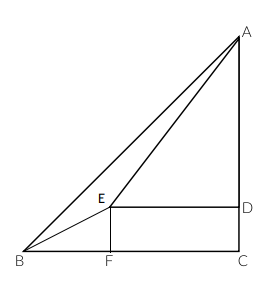Question 109

# At the foot of the mountain, the angle of elevation of the summit at the top of the mountain is 45°. After ascending 100 metres, at a slope of 30° up the mountain towards the summit. the angle of elevation of the summit is 60°. Find the height of the summit.

SolutionLet the point intersecting inside triangle ABC be 'E'.
It is given, $$\ \angle\ AED$$ = 60, $$\ \angle\ ABC$$ = 45, $$\ \angle\ EBC$$ = 30 and BE = 100
At point E, $$\ \angle\ BEF\ =60,\ \angle\ DEF=90\ and\ \angle\ AED\ =\ 60$$
$$\ \angle\ AEB\ =\ 360\ -\ 60\ -\ 60\ -\ 90\ =\ 150$$
In triangle AEB, $$\ \angle\ EAB\ =\ 180\ -\ 150\ -\ 15\ =\ 15$$
$$\ \angle\ EAB\ =\angle\ EBA$$
Therefore, EA = 100 (as BE = 100)
EF = DC = 50
In triangle AED,
$$ED^2+AD^2=AE^2$$
ED = x, AD = $$\sqrt{\ 3}$$x
$$4x^2=\left(100\right)^2$$
2x = 100
x = 50
Height of summit = $$\sqrt{\ 3}x\ +\ 50$$ = $$50\left(\sqrt{\ 3}+1\right)$$